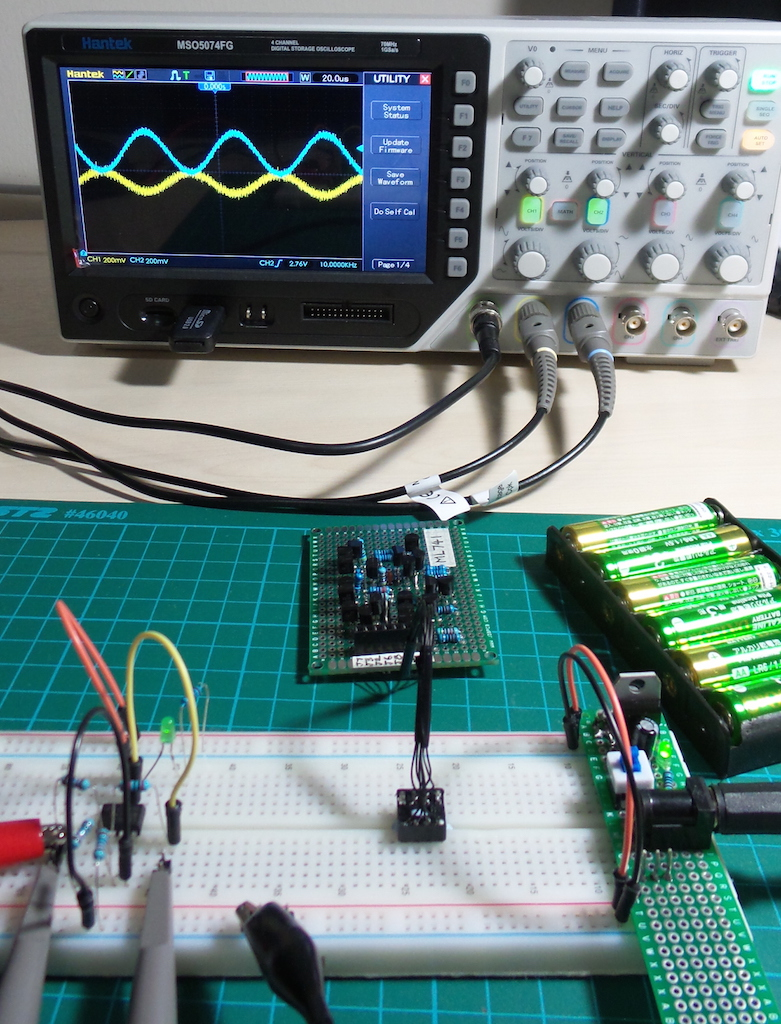# #159 ML741/InvertingAmplifier

Test an inverting amplifier circuit using the ML741 discrete component opamp

This is a demonstration of an inverting amplifier circuit using the ML741 discrete component opamp.

The inverting amplifier is a generalised case of the basic Inverter circuit, but with an arbitrary gain determined by the input and feedback resistor selection:

``````Vout = - Rf/Rin * Vin
``````### How it works

Fundamentally, an op-amp strives to keep its inverting an non-inverting inputs equal by modulating the output.

In the inverter/inverting amplifier configuration, the inverting input is at the inflexion point of the Rin:Rf voltage divider. Hence the op amp achieves inverting/non-inverting input equilibrium when `Vout = - Rf/Rin * Vin`.

## Construction

In this circuit, I am using a single rail supply (V- = GND) instead of the “conventional” dual rail supply (V+/V-).

For this reason, the non-inverting input is pegged to V+/2 with a voltage divider. In a dual rail configuration, V+/2 is usually “ground”.

The breadboard build has a fixed gain of -2 but of course the feedback resistor can be replaced with a pot for manual variable gain.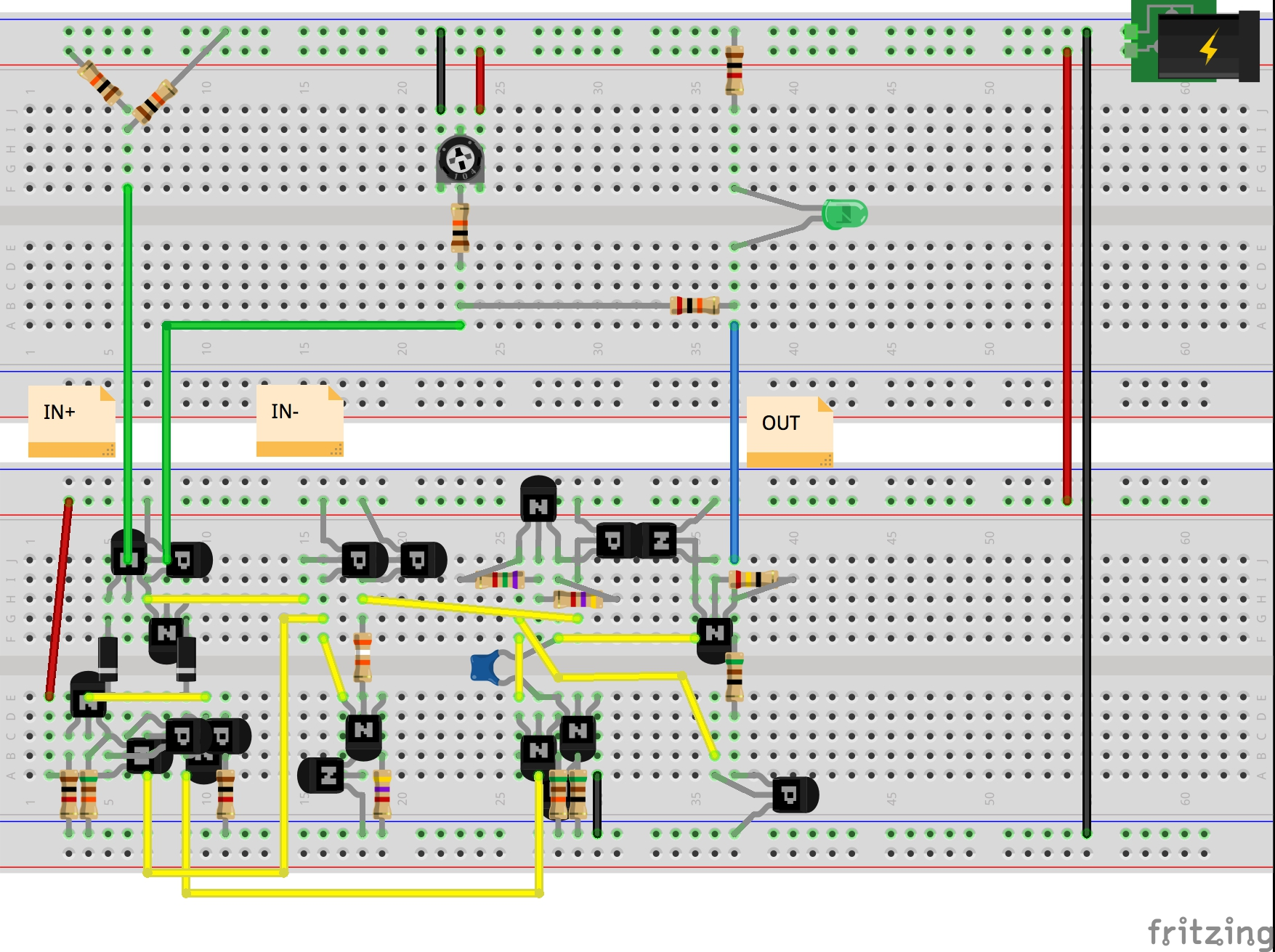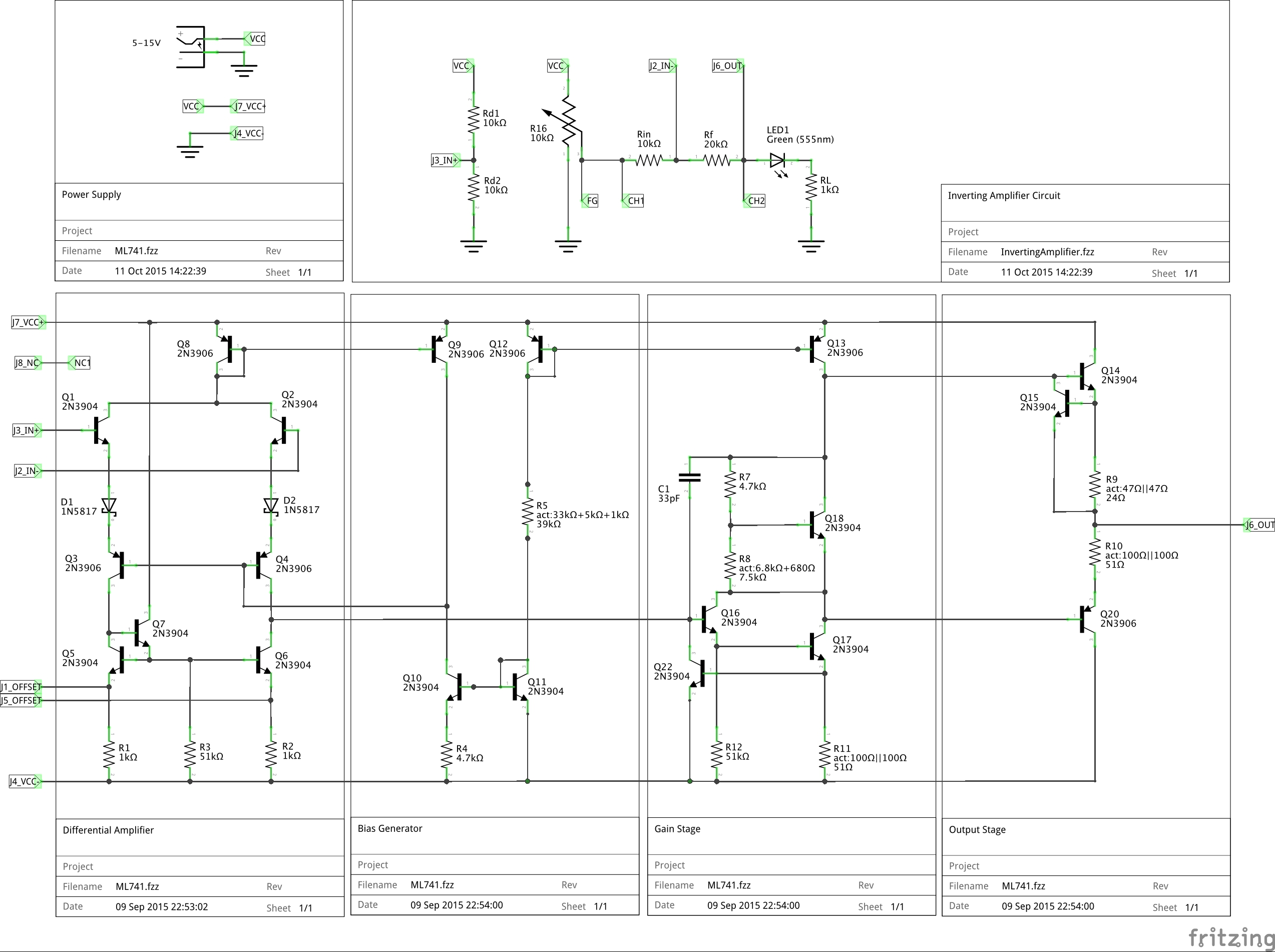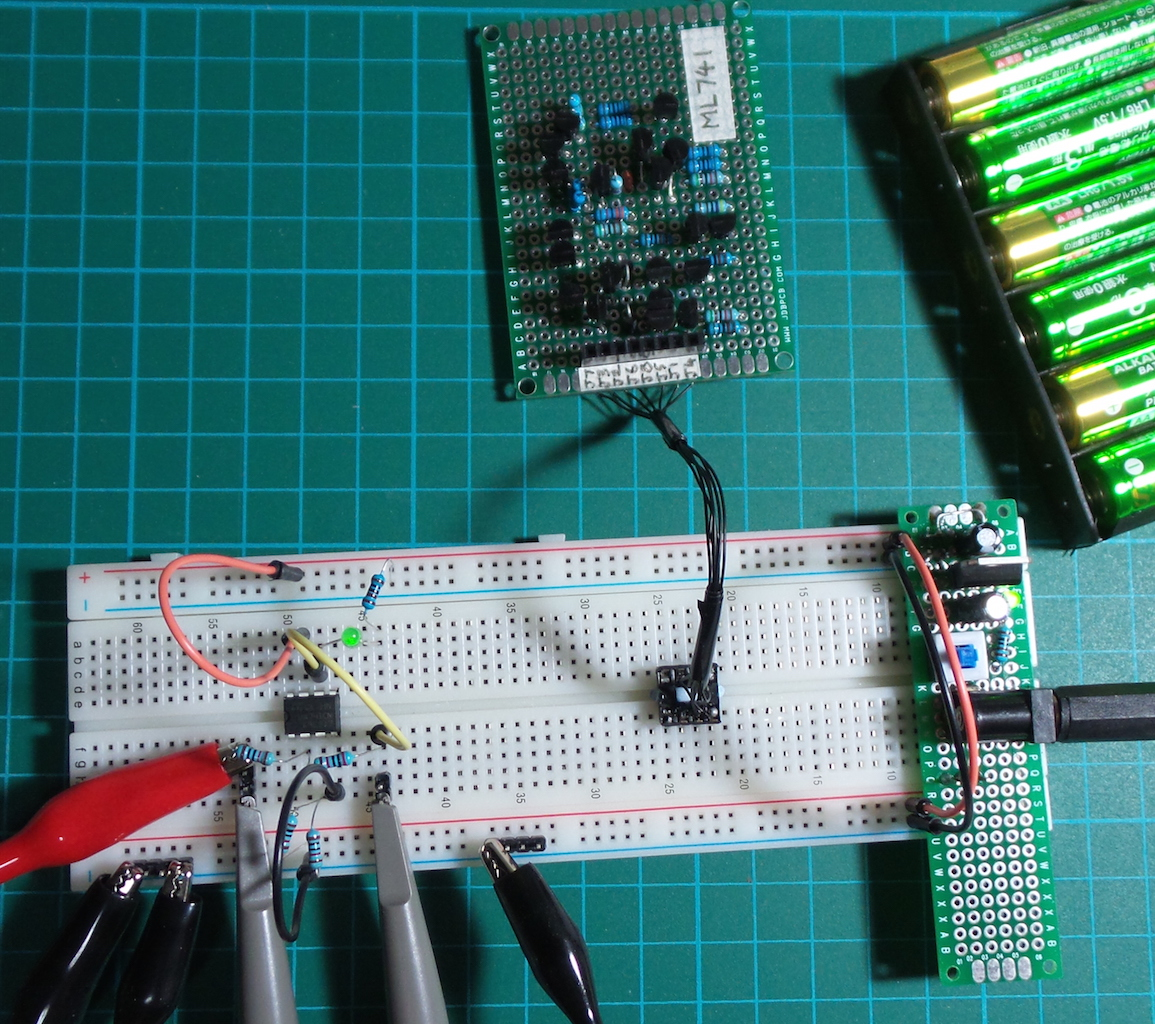## ML741 v “real” 741 Test

Here are some results comparing the behaviour of a standard UA741CN chip with the ML741 (protoboard version).

Setup:

• power is 5V single rail, i.e. V- = GND
• non-inverting input is fed a sine wave 200mVpp with 2.5V DC offset
• the function generator sine wave input replaces the manual 10kΩ input pot in the schematic above (at node FG)
• CH1 and CH2 signals are DC coupled and vertical shifted by -2.5V in the screenshots that follow

Scope connections

• CH1: non-inverting input
• CH2: output

### At 10kHz

• very slight phase shift for both opamps
• UA741CN is delivering almost exactly -2x gain
• ML741 is also generating -2x gain, but the output is DC shifted up by ~20mV

ML741: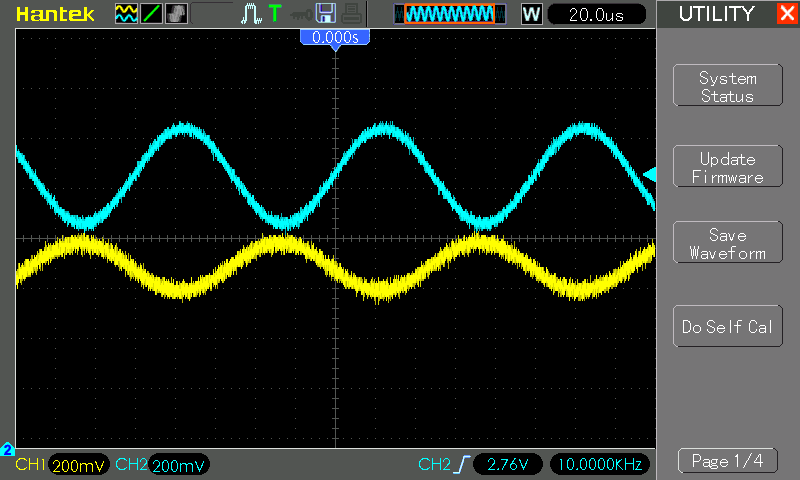UA741CN: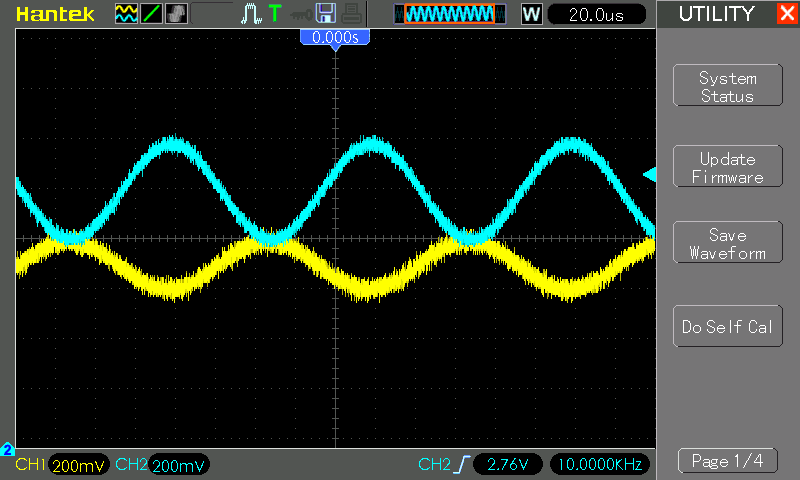### At 100kHz

• phase shift has increased for both opamps
• output has started to attenuate. By 150kHz or so, already hitting unit gain
• ML741 output remains pulled higher by ~20mV

ML741: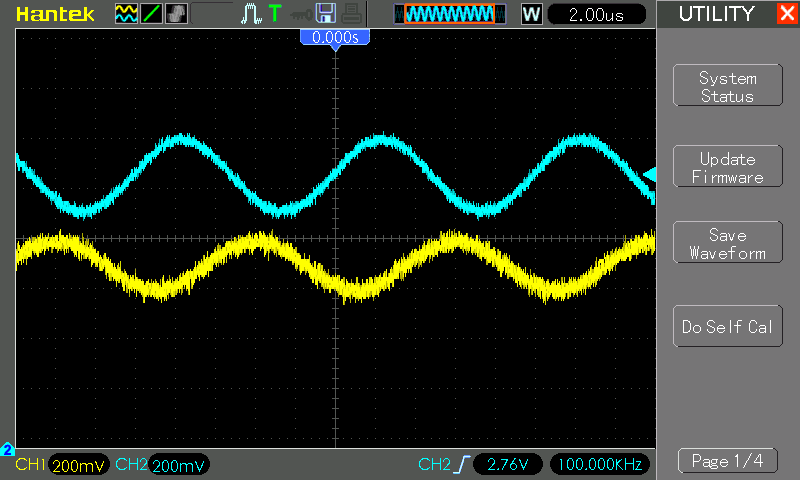UA741CN: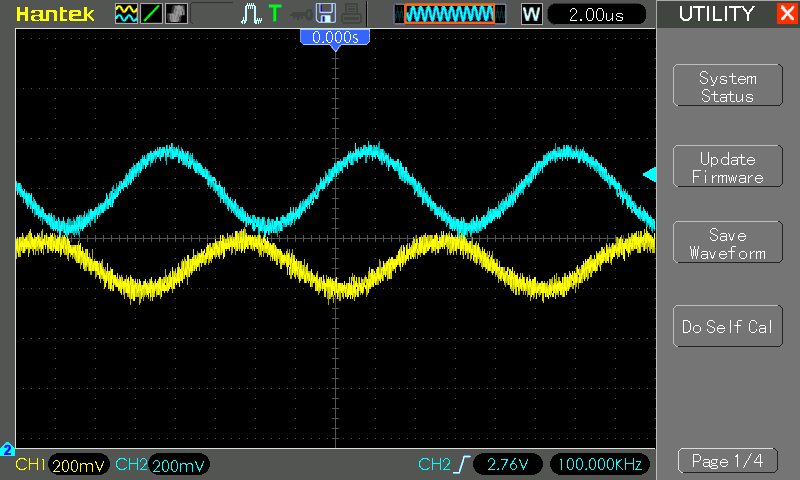Measurements in action…